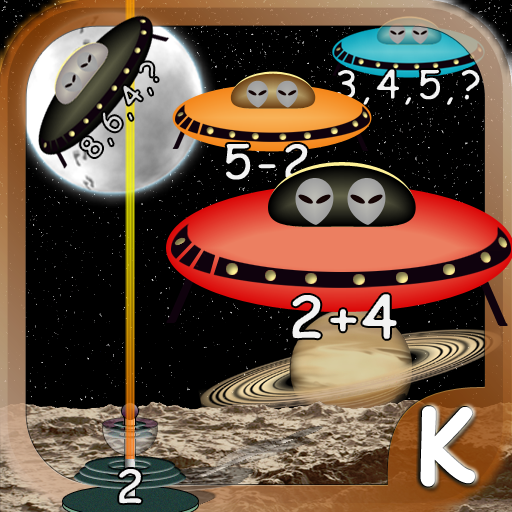@Reks: Educational iOS Applications

## 2nd Grade Printable Math Quiz Workshe...You can download a number of math practice worksheets designed for Grade 2  Elementary School. The practice worksheets are based on Math Essentials textbook and math common core standards. Unit 1 covers the following concepts: add and subtract numbers up to 20 add three or four numbers find partners and totals write equations for math mountains tell […]* Three counting up games  * Two counting down games * Two addition games * One subtraction game * Basic facts about the Solar System * Focus on Math problems to avoid random shoot-out * Complete missions to move to the next level and earn space pilot ranks * Collect insignia * Apply fine motor […]